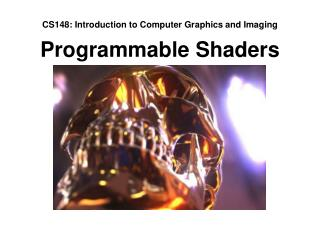# CS148: Introduction to Computer Graphics and Imaging Programmable Shaders - PowerPoint PPT PresentationDownload PresentationCS148: Introduction to Computer Graphics and Imaging Programmable Shaders

CS148: Introduction to Computer Graphics and Imaging Programmable Shaders
Download Presentation## CS148: Introduction to Computer Graphics and Imaging Programmable Shaders

- - - - - - - - - - - - - - - - - - - - - - - - - - - E N D - - - - - - - - - - - - - - - - - - - - - - - - - - -
##### Presentation Transcript

1. CS148: Introduction to Computer Graphics and Imaging Programmable Shaders

2. Topics The Graphics Pipeline • Fixed-function • Programmable Programming Model • Vertex, Geometry, Pixel Shaders • Parallelism Implementation • GLSL Syntax • Limitations GPGPU

3. The Graphics Pipeline

4. Geometry Rendering Stages Vertex Data Tessellation Vertex Processing Pixel Processing Geometry Processing Pixel Rendering Primitive Data Texture Sampler Textured Surface

5. Rasterization Input: Vertices & values (ex. color, texture) Output: Pixels & interpolated values

6. Fixed Function Pipeline Math OpenGL glLightfv(GL_LIGHT0, GL_DIFFUSE, P); glLightfv(GL_LIGHT0, GL_POSITION, C); glEnable(GL_LIGHT0); glBlendFunc(GL_SRC_ALPHA, GL_ONE); glEnable(GL_BLEND); ?

7. Programmable Rendering Code Vector4 RenderPixel( Vector3 Position, Vector3 Normal) { ... return Vector4(0, 0, 1, 0); }

8. Limitations Mutable Pixels Geometry Vertices Immutable • Clipping • Triangle scanlines

9. Direct3D 10 pipeline • Traditional graphics pipeline • Three application-programmable stages operate on independent vertex, primitive, and pixel fragments • All stages share the same programming model and can query the same resources Vertices Input Assembler Textures Sampler Vertex Shader Constants Textures Sampler Geometry Shader Constants CPU or GPU Memory Rasterizer Textures Sampler Pixel Shader Constants Application Programmable Target Surface Output Merger

10. Programming Model

11. CPU vs. GPU programming

12. Vertex Shader Inputs • Program-provided vertex data • glVertex3d(1.0, 2.0, 3.0); • glNormal3d(1.0, 0.0, 0.0); • Constants • Textures • Outputs • Position • Array of floats used by future pipeline stages • Structure determined entirely by programmer

14. Geometry Shader Inputs • Geometric primitive (point, line, triangle, quad) • Adjacent vertex information (AV0, AV1, AV2) • Outputs • More geometric primitives

15. Pixel Shader Inputs • Array of floats from vertex/geometry shader • Constants • Textures • Outputs • 4-channel color • Depth

17. Implementation

18. Simple Vertex Shader void main() { gl_Position = gl_ModelViewProjectionMatrix * gl_Vertex; } gl_Position = output of vertex shader gl_Vertex = glVertex3d(x, y, z); or STShape::… gl_ModelViewProjectionMatrix = glLoadMatrixd(M); or STTransform::…

19. Variables Local Variables { vec2 SomeVector = vec2(sin(2.0), sqrt(3.0)); float SomeFloat = SomeVector.x * SomeVector.y; } “Varying” Variables Varyings are output by the vertex shader and interpolated across each primitive for the pixel shader varying vec2 TextureCoord0; void main() { TextureCoord0 = vec2(gl_Position.x, 2.0); }

20. Variables Uniform Variables Uniforms are variables set by the program that can be changed at runtime, but are constant across each execution of the shader uniform float CurrentTime; void main() { float Displacement = cos(CurrentTime * 10); } C++ code to change this value might look like: STVertexShader Shader; Shader.SetUniformVariable(“CurrentTime”, ElapsedTimeInSeconds);

21. Textures Textures are mapped from (0, 0) to (1, 1) Only relatively recent cards support vertex shader textures, almost all support pixel shader textures uniform sampler2D SomeTexture; void main() { vec4SomeTextureColor = texture2D(SomeTexture, vec2(0.5, 0.5)); }

22. Vectors Constructors vec3 V3 = vec3(1.0, 2.0, 3.0); vec4 V4 = vec4(V3, 4.0); Swizzling vec2 V2 = V4.xy; vec4 V4Reverse = V4.wzyx; vec4 Result = V4.xyzw + V4.xxxx; Basic Vector Operators float Result = dot(V4, V4Reverse); vec3 Result = cross(V3, vec3(1.0, 0.0, 0.0));

23. Simple Pixel Shader varying vec2 TexCoord0; varying vec2 TexCoord1; uniform sampler2D SomeTexture0; uniform sampler2D SomeTexture1; void main() { gl_FragColor = texture2D(SomeTexture0, TexCoord0) * 0.5 + texture2D(SomeTexture1, TexCoord1) * 0.5; } gl_FragColor = output of pixel shader

24. Functions Very similar to C: boolCompare(in vec3 a, in vec3 b) {return dot(a, a) > dot(b, b); }

25. Limitations Memory • No access to nearby pixels • Cannot bind render target as input texture • Limited stack space, instruction count • Performance • Branching support is limited and slow • Graphics card will timeout if code takes too long • Variable support across different graphics cards • Programmable code is much slower than dedicated hardware

26. Practical Shading: The Bloom Effect Images from Gamasutra.com & Tron 2.0

27. Practical Shading: Bump-mapping A fraction of the cost of increasing the initial number of polygons or using the geometry shader

28. GPGPU

29. Parallelism CPU • Thread synchronization • Bandwidth limitations • Scheduling overhead • Small number of cores GPU • All threads independent • Message passing is not allowed • Resource allocation is done by GPU • Many cores available (12 to 48 typical)

30. Ideal GPU Problems GPU’s are great if the problem: • Executes the same code many times on different input • Needs lots of math units at the same time • Does not share data between executing components • Can be expressed as a sequence of operations acting on a stream of data • Large data sets • Has lots of work to do without CPU intervention

31. General Purpose GPU’s Beyond triangle rendering • Collision detection • Fluid simulation • Physics • Raytracing • Video compression • Beyond graphics • Folding@Home • Speech Recognition • Partial differential equation solvers • Fourier transform

32. Object Reconstruction from Images Massive non-linear optimization problem Output: Geometry of photographed object Input: 200 640x480 images (~250MB)